# GSEB Solutions Class 7 Maths Chapter 5 Lines and Angles Ex 5.1

Gujarat Board GSEB Textbook Solutions Class 7 Maths Chapter 5 Lines and Angles Ex 5.1 Textbook Questions and Answers.

## Gujarat Board Textbook Solutions Class 7 Maths Chapter 5 Lines and Angles Ex 5.1Question 1.
Find the complement of each of the following angles: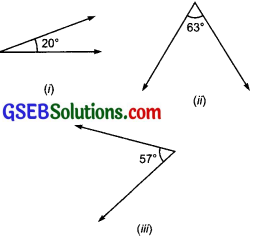Solution:
(i) Complement of 20° = 90° – 20° = 70°
(ii) Complement of 63° = 90° – 63° = 27°
(iii) Complement of 57° = 90° – 57° = 33°

Question 2.
Find the supplement of each of the following angles: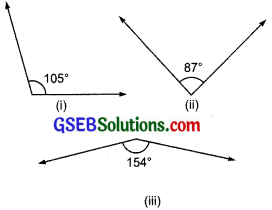Solution:
(i) Supplement of 105° = 180° – 105° = 75°
(ii) Supplement of 87° = 180° – 87° = 93°
(iii) Supplement of 154° = 180° – 154° = 26°

Question 3.
Identify which of the following pairs of angles are complementary and which are supplementary.
(i) 65°, 115°
(ii) 63°, 27°
(iii) 112°, 68°
(iv) 130°, 50°
(v) 45°, 45°
(vi) 80°, 10°
Solution:
(i) ∵ 65° + 115° = 180°
∴ 65° and 115° are supplementary angles.

(ii) ∵ 63° + 27° = 90°
∴ 63° and 27° are complementary angles.

(iii) ∵ 112° + 68° = 180°
∴ 112° and 68° are supplementary angles.

(iv) ∵ 130° + 50° = 180°
∴ 130° and 50° are supplementary angles

(v) ∵ 45° + 45° = 90°
∴ 45° and 45° are complementary angles.

(vi) ∵ 80° + 10° = 90°
∴ 80° and 10° are complementary angles.

Question 4.
Find the angle which is equal to its complement.
Solution:
Let the required angle be x.
∵ It is equal to its complement,
∴ x = 90° – x
[∵ (90° – x) is complement of x] or x + x = 90°
[Transposing x from R.H.S. to L.H.S.]
or 2x = 90°
Dividing both sides by 2, we have
$$\frac { 2x }{ 2 }$$ = $$\frac { 90° }{ 2 }$$ or x = 45°
Thus, 45° is equal to its complement.

Question 5.
Find the angle which is equal to its supplement.
Solution:
Let the required angle be m and supplement of m = (180° – m)
∵ m is equal to its supplement.
∴ m = 180° – m
or m + m = 180°
[Transposing m from R.H.S. to L.H.S.]
or 2m = 180°
Dividing both sides by 2, we have
$$\frac { 2m }{ 2 }$$ = $$\frac { 180° }{ 2 }$$ or m = 90°
Thus, 90° is equal to its supplement.

Question 6.
In the given figure, ∠1 and ∠2 are supplementary angles. If ∠1 is decreased, what changes should take place in ∠2 so that both the angles still remain supplementary.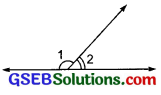Solution:
In case ∠1 is decreased, the same amount of degree measure is added to ∠2, i.e. ∠2 be increased by same amount of degree measure.

Question 7.
Can two angles be supplementary if both of them are:
(i) acute?
(ii) obtuse
(iii) right?
Solution:
(i) ∵ Sum of two acute angles is always less than 180°.
∴ Two acute angles cannot be supplementary.

(ii) ∵ Sum of two obtuse angles is always more than 180°.
∴ Two obtuse angles cannot be supplementary.

(iii) ∵ Sum of two right angles = 180°.
∴ Two right angles are supplementary.

Question 8.
An angle is greater than 45°. Is its complementary angle greater than 45° or equal to 45° or less than 45″?
Solution:
Complement of an angle (greater than 45°) is less than 45°.

Question 9.
(i) Is ∠1 adjacent to ∠2?
(ii) Is ∠AOC adjacent to ∠AOE?
(iii) Do ∠COE and ∠EOD form a linear pair?
(iv) Are ∠BOD and ∠DOA supplementary?
(v) Is ∠1 vertically opposite to ∠4?
(vi) Which is the vertically opposite angle of ∠5?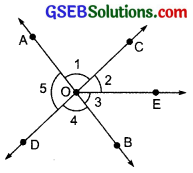Solution:
(i) Yes, ∠1 and ∠2 are adjacent angles. Yes because both the angles have common arm OC and common vertex O.
(ii) No, ∠AOC is not adjacent to ∠AOE, because ∠AOC is part of ∠AOE.
(iii) Yes, ∠COE and ∠EOD form a linear pair, because $$\overset { \longleftrightarrow }{ COD }$$ is a straight line.
(iv) Yes, ∠BOD and ∠DOA are supplementary, because ∠BOD + ∠DOA = 180°.
(v) Yes, because AB and CD are straight lines.
(vi) The vertically opposite Wangle of ∠5 is ∠BOC (or ∠COB).

Question 10.
Indicate which pairs of angles are:
(i) Vertically opposite angles.
(ii) Linear pairs.
Solution:
(i) Vertically opposite angles:
In the figure, following pairs are vertically opposite angles:
∠1 and ∠4
∠5 and (∠2 + ∠3)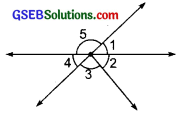(ii) Linear pairs:
∠4 and ∠5 form a linear pair.
∠1 and ∠5 form a linear pair.
∠1 and (∠3 + ∠2) form a linear pair.
∠4 and (∠3 + ∠2) form a linear pair.

Question 11.
Solution:
No, ∠1 and ∠2 are not adjacent angles because they do not have a common vertex.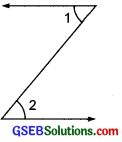Question 12.
Find the value of the angles x, y and z in each of the following: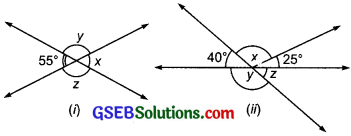Solution:
(i) Since x and 55° are vertically opposite angles
x = 55°
Again, 55° + y = 180° [Linear pair]
or y = 180° – 55°
or y = 125°
Since z and y are vertically opposite angles,
∴ z = 125° [∵ y = 125°]
Thus, x = 55°, y = 125°, z = 125°

(ii) Since 40° and z are vertically opposite angles, ∴ z = 40°
Again y and 40° form a linear pair.
∴ y + 40° = 180°
[Transposing 40° to R.H.S.]
or y = 180° – 40°
or y = 140°
∵ y and (x + 25°) are vertically opposite angles
∴ (x + 25°) = y = 140° [∵ y = 140°]
or x = 140° – 25°
[Transposing 25° to R.H.S.]
or x = 115°
Thus, x = 115°, y = 140° and z = 40°

Question 13.
Fill in the blanks:
(i) If two angles are complementary, then the sum of their measures is ______.
(ii) If two angles are supplementary, then the sum of their measures is ______.
(iii) Two angles forming a linear pair are ______.
(iv) If two adjacent angles are supplementary, they form a ______.
(v) If two lines intersect at a point, then the vertically opposite angles are always ______.
(vi) If two lines intersect at a point, and if one pair of vertically opposite angles are acute angles, then the other pair of vertically opposite angles are ______.
Solution:
(i) 90°
(ii) 180°
(iii) supplementary
(iv) linear pair
(v) equal
(vi) obtuse angles.

Question 14.
In the adjoining figure, name the following pairs of angles: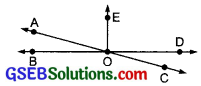(i) Obtuse vertically opposite angles.